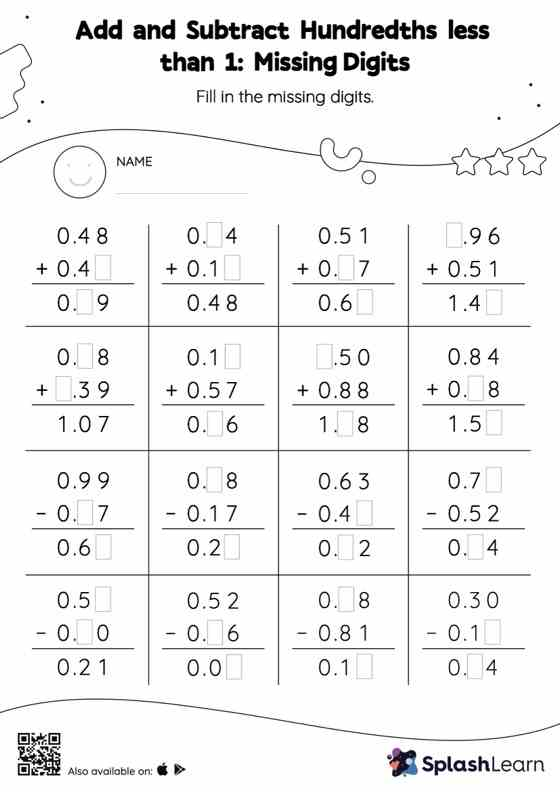# Add and Subtract Hundredths less than 1: Missing Digits Worksheet

Home > Add and Subtract Hundredths less than 1: Missing DigitsThis worksheet gives students an opportunity to apply the concepts of addition and subtraction to add and subtract hundredths less than 1. Students align the decimal points and use zero as space holders to add and subtract decimals. Then they use the relationship between addition and subtraction to find the missing number in add and subtract hundredths less than 1 worksheet. In each problem, the numbers are laid out in the vertical format. In this format, pair of digits in each successive place are tackled one by one. This helps students follow a structured approach.